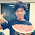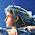[C#][Visual Studio] 控制流程 Control Flow

2017-08-20

介紹

if else 敘述式

if - else 為我們判斷敘述式，以下列程式為例：
1. 當變數 input 大於 0 的時候， classify 內容被指定為 positive
2. 當變數 input 等於 0 的時候， classify 內容被指定為 0
3. 當變數 input 不符合上述所有條件的時候， classify 內容被指定為 negative
```if (input > 0)
{
classify = "positive"
}
else if (input == 0)
{
classify = "zero";
}
else
{
classify = "negative";
}
```

(X)
if (input > 0)
classify = "positive";
(O)
if (input > 0)
{
classify = "positive";
}

```if (input > 0)
{
classify = "positive";
}
else
{
classify = "negative";
}
```

`classify = (input > 0) ? "positive" : "negative";`

```int? x = null;
int y = x ?? -1;
```
(O)處理 null 是一件相當耗費人力與效能的事情，無論在程式或資料庫，請盡可能不要讓您的資料、變數可為 null。

switch 判斷式

switch 也是判斷式之一，依據不同的情境案例，進行不同的程式運算，可以用來取代 if-else判斷式。如下列程式為例，整數變數 case 若為1，則印出 "Case 1"於銀幕上；若為 2，則印出 "Case 2"；若皆不符合條件，則印出 "Default"。
```int choice = 1;

switch (choice)
{
case 1:
Console.WriteLine("Case 1");
break;
case 2:
Console.WriteLine("Case 2");
break;
default:
Console.WriteLine("Default");
break;
}
```

for 迴圈

```for (int i = 0; i < 10; i++)
{
Console.WriteLine("this is a loop !!");
}
```

```string[] score = {"63", "70", "50", "88", "77", "65", "100", "75", "77", "70"};
for (int i = 0; i < score.Length; i++)
{
Console.WriteLine(score[i]);
}
```

foreach 迴圈

```List<string> score = new List<string>();

foreach(var item in score)
{
Console.WriteLine(item);
}
```

while 與 do while

```int n = 1;
while (n < 6)
{
Console.WriteLine("Current value of n is {0}", n);
n++;
}
```
```int x = 0;
do<
{
Console.WriteLine(x);
x++;
} while (x < 5);
```

break 與 continue

break 與 continue 為跳躍指令。以迴圈為例：當迴圈程式執行到這兩個指令，會進行跳出迴圈與持續下一迴圈的操作行為。
```for (int i = 1; i <= 100; i++)
{
if (i == 3)
{
break;
}
Console.WriteLine(i);
}```
// 輸出
1
2

```for (int i = 1; i <= 10; i++)
{
if (i < 5)
{
continue;
}
Console.WriteLine(i);
}```
//輸出
6
7
8
9
10

參考資料

1. Study4TW 內部教材
2. ?: 運算子 (C# 參考) - Microsoft docs
2. ?? 運算子 (C# 參考) - Microsoft docs

{ 9 意見... read them below or Comment }

1.那個...這一段你寫錯了，stringArray是不需要new string的，這樣才對。
string[] score = {"63", "70", "50", "88", "77", "65", "100", "75", "77", "70"};
for (int i = 0; i < score.count ; i++)
{
Console.WriteLine(score[i]);
}

1.感謝提醒，
寫到昏頭忘記確認寫程式與法，已修正 :) 。

2.int case = 1;

switch (case)
{
case 1:
Console.WriteLine("Case 1");
break;
case 2:
Console.WriteLine("Case 2");
break;
default:
Console.WriteLine("Default");
break;
}

case沒辦法拿來當變數名稱，需要用case1才行，不然無法執行

3.感謝提醒，我真是太不嚴謹了。
已經修正，謝謝您 :)

2.好讚阿～謝謝尼0w0

3.對於初學者,
謝謝有你的文章QwQ~~

4.謝謝啦OWO!!!

5.我不太懂程式 正在學c#，如有冒犯請見諒。
想問下其中您文章提到﹝若為 2，則印出 "Case 1"﹞是否應該是印出Case 2 ?
謝謝

1.你是對的，這邊筆誤。
感謝提醒